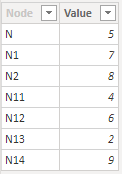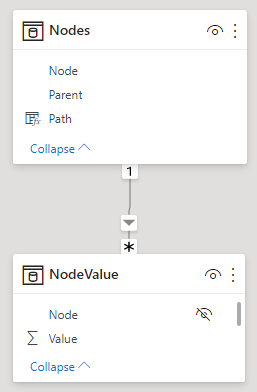cancel
Showing results for
Did you mean:New Member

## How to make hierarchical path use visual filters

Hi everyone,

I have a table which contains some hierarchical data with id and parentid fields among others fields.

I can create appropriate structure with PATH (and other path related DAX functions). I can also display it visually with level hierarchy in Hierarchy Slicer.

Each row in this table additionally has certain numeric field. What I need to determine is the value of this field for the top-most selected (not filtered-out) parent leaf for each row.

So, for example, for tree like this with this significant numeric value written in parentheses next to node names:

N(5)

/  \

N1(7)                  N2(8)

/  \                      /   \

N11(4)  N12(6)     N13(2)   N14(9)

If all nodes are selected in a slicer, I need to get value (5) for all rows (in some new measure or column) as the value, since 5 is the field value of top-most parent.

If N1, N11 and N12 are the only ones selected then all three of them should have (7) as a value for this new field.

If on the other hand N13 is the only one selected then it should have (2) as a value for this field.

I am able to acchieve this with PATH related functions but I cannot make it work with filters applied. It always works on an entire dataset and always returns topmost value (5) for all rows.

P.S. This should also work with URL filtering when table rows are filtered by external URL parameters when embedding powerBI report in a web page.

Any help is appreciated. Thank you very much.

1 ACCEPTED SOLUTIONSuper User

Here's an initial idea - PBIX attached.

I'm working on the assumption that you have a data model with:

1. Nodes table, with columns Node, Parent and Path (created using PATHITEM)
2. NodeValue table containing Node and Value columns.
3. Relationship between NodeValue[Node] and Nodes[Node]``````Path =
PATH ( Nodes[Node], Nodes[Parent] )``````

Then you can create a measure like the one below (note that this can most likely be optimized).

Assumptions I made in writing the measure:

• You only want the measure evaluated for a single node at a time.
• The measure calculates the value sum for the topmost node in the path for the currently filtered node, within the overall selection of nodes (using ALLSELECTED).

``````Topmost node value =
VAR CurrentNode =
SELECTEDVALUE ( Nodes[Node] )
RETURN
IF (
NOT ISBLANK ( CurrentNode ),
-- Only return result if single node selected
VAR CurrentNodePath =
-- Get path of current node
SELECTEDVALUE ( Nodes[Path] )
VAR CurrentNodePathLength =
PATHLENGTH ( CurrentNodePath )
VAR CurrentNodePathTable =
-- Table containing Node/Position pairs for CurrentNodePath
SELECTCOLUMNS (
GENERATESERIES ( 1, CurrentNodePathLength ),
-- Table of values {1,2,...,CurrentNodePathLength}
"@Node", PATHITEM ( CurrentNodePath, [Value] ),
"@Position", [Value]
)
VAR VisibleNodesAllSelected =
-- List of all nodes selected overall
CALCULATETABLE (
VALUES ( Nodes[Node] ),
ALLSELECTED ()
)
VAR CurrentNodePathTableFiltered =
-- Filter path to just those nodes selected overall
FILTER (
CurrentNodePathTable,
[@Node] IN VisibleNodesAllSelected
)
VAR TopNode =
-- Get the top Node (i.e. with minimum position in the path)
-- and treat as Nodes[Node] value
TREATAS (
SELECTCOLUMNS (
TOPN ( 1, CurrentNodePathTableFiltered, [@Position], ASC ),
"@TopNode", [@Node]
),
Nodes[Node]
)
RETURN
CALCULATE ( [Value Sum], TopNode, ALL ( Nodes ) )
)``````

For example, if you select N1, N11, N12, N2, N14, then:

• N1, N11 and N12 have Topmost node value = 7
• N2 and N14 have Topmost node value = 8Hopefully this is of some use and can be adapted to your model.

Regards,

Owen

Owen Auger
Blog
5 REPLIES 5New Member

@OwenAuger  Thank you for your help so far. Just a quick question.

If I had a new (child) table to the Node table - let's call it a Leaf table. It has foreign key to node_id of course among other fields. Any Node can have any number of Leaves.

If I needed to display the Topmost node measure value in the visual that displays the Leaves data of selected Nodes, I suppose that the measure would need to be changed in a way that SELECTEDVALUE is no longer the way to get the current node for the Leaf. I should somehow use the Node from the filter context of the Leaf.

Is that correct way of thinking? Thank you.Super User

You're welcome! 🙂
I just managed to get back to looking at this.

The exact way of handling this depends on your data model setup.

I have attached an updated PBIX using option 1 below, with a NodeChild table added.

1. You could set up the model with the NodeChild table having a bidirectional relationship with the Node table. This would mean the filter on a Child would propogate to the corresponding node.

There would just be a small tweak required to the measure near the end, changing ALL ( Nodes ) to ALLCROSSFILTERED ( Nodes ). This is because we need to remove all filters affecting the Nodes table, including those on the many-side of a relationship with Nodes.

I have used this method in the attached PBIX.

2. If you don't have a bidirectional relationship between Nodes and NodeChild, then you need to use DAX to filter Nodes based on NodeChild. There are different ways to do this, but one is to wrap the SELECTEDVALUE calls in CALCULATE with NodeChild as a filter argument, so you end up with code like below:
``````VAR CurrentNode =
CALCULATE (
SELECTEDVALUE ( Nodes[Node] ),
NodeChild
)

VAR CurrentNodePath =
CALCULATE (
SELECTEDVALUE ( Nodes[Path] ),
NodeChild
)``````

Hope this helps!

Regards,

Owen

Owen Auger
BlogNew Member

Thank you!New Member

Hey Owen,

Thank you so much, that solved the problem. I lost two days jumping around it. You rock! 🙂

Best,

D. SenkovicSuper User

Here's an initial idea - PBIX attached.

I'm working on the assumption that you have a data model with:

1. Nodes table, with columns Node, Parent and Path (created using PATHITEM)
2. NodeValue table containing Node and Value columns.
3. Relationship between NodeValue[Node] and Nodes[Node]``````Path =
PATH ( Nodes[Node], Nodes[Parent] )``````

Then you can create a measure like the one below (note that this can most likely be optimized).

Assumptions I made in writing the measure:

• You only want the measure evaluated for a single node at a time.
• The measure calculates the value sum for the topmost node in the path for the currently filtered node, within the overall selection of nodes (using ALLSELECTED).

``````Topmost node value =
VAR CurrentNode =
SELECTEDVALUE ( Nodes[Node] )
RETURN
IF (
NOT ISBLANK ( CurrentNode ),
-- Only return result if single node selected
VAR CurrentNodePath =
-- Get path of current node
SELECTEDVALUE ( Nodes[Path] )
VAR CurrentNodePathLength =
PATHLENGTH ( CurrentNodePath )
VAR CurrentNodePathTable =
-- Table containing Node/Position pairs for CurrentNodePath
SELECTCOLUMNS (
GENERATESERIES ( 1, CurrentNodePathLength ),
-- Table of values {1,2,...,CurrentNodePathLength}
"@Node", PATHITEM ( CurrentNodePath, [Value] ),
"@Position", [Value]
)
VAR VisibleNodesAllSelected =
-- List of all nodes selected overall
CALCULATETABLE (
VALUES ( Nodes[Node] ),
ALLSELECTED ()
)
VAR CurrentNodePathTableFiltered =
-- Filter path to just those nodes selected overall
FILTER (
CurrentNodePathTable,
[@Node] IN VisibleNodesAllSelected
)
VAR TopNode =
-- Get the top Node (i.e. with minimum position in the path)
-- and treat as Nodes[Node] value
TREATAS (
SELECTCOLUMNS (
TOPN ( 1, CurrentNodePathTableFiltered, [@Position], ASC ),
"@TopNode", [@Node]
),
Nodes[Node]
)
RETURN
CALCULATE ( [Value Sum], TopNode, ALL ( Nodes ) )
)``````

For example, if you select N1, N11, N12, N2, N14, then:

• N1, N11 and N12 have Topmost node value = 7
• N2 and N14 have Topmost node value = 8Hopefully this is of some use and can be adapted to your model.

Regards,

Owen

Owen Auger